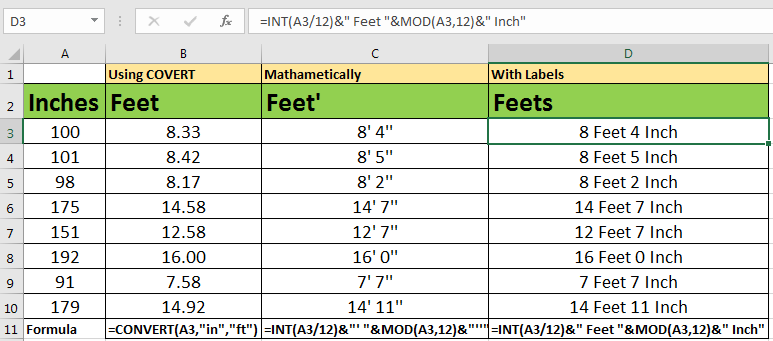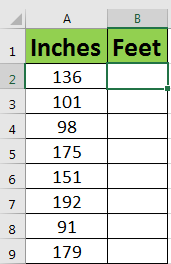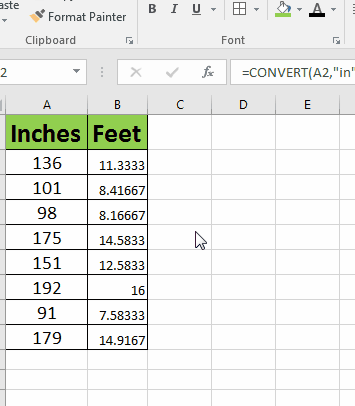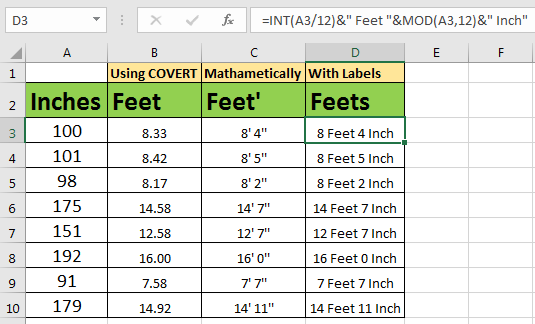# How to Inches to Feet in Excel

In Excel, there is a function named CONVERT that can be used to create inch to feet converter in excel. There are other methods too to make a inches to feet converter. In this article we will explore them all.How to Create a Inches To Feet Converter?
So through out the article we will have below sample data.Using CONVERT function

In excel 2016, there is this function COVERT that is used to convert almost any measurement to another. In this article we will use it convert Inches to Feet.

Generic Formula for Conversion of Inches to Ft

=CONVERT(number,”in”,”ft”)

Number: the number of inches that you want to convert into feet.
“In”: initial for inches. To tell function that input number in inches.
“Ft”: initial for Feet. To tell function that output needs to be feet.

Now to convert above inches into feets write this conversion formula in cell B2 and drag it down.

=CONVERT(A2,"in","ft")In the above gif you can see there are too many options are available for conversion.

In this example, we got our feets in decimal. The whole part is feet and decimal part is inches. But sometimes you would want to have Ft’ In’’ format (e.g. 6’ 1”). Let’s see how can we do this.
Convert Inches to Feet With Format
Now we will convert Inches into Feets mathematically. So as you know that 12’’ inches are equal to 1’ 00’’ feet. So to convert any number of inches we need to divide it by 12 get its integer part. And then concatenate with reminder.

Generic Formula

=INT(number/12)&”’ “&MOD(number,12)&”’’”

The INT function will get integer part only of number when divided by 12. MOD function will get Reminder of number when divided by 12. The number is inches. It can be in a cell reference.

So to implement this formula write below formula in cell C2 and drag it down.

=INT(A2/12)&"' "&MOD(A2,12)&"''"Here we concatenated ‘ and “ with feet and inch part. If you want complete labels like 6 feet 2 inch then you can replace ‘ and “ with feet and inch respectively. See below formula.

=INT(A3/12)&" Feet "&MOD(A3,12)&" Inch"Popular Articles:

50 Excel Shortcuts to Increase Your Productivity

How to use the VLOOKUP Function in Excel

How to use the COUNTIF function in Excel

How to use the SUMIF Function in Excel

1.